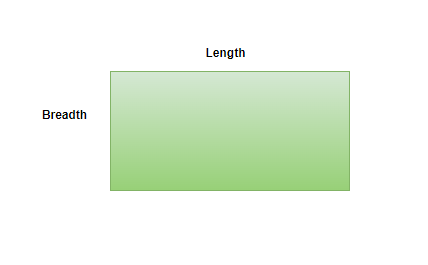# Perimeter of Rectangle Formula

• Last Updated : 29 Dec, 2021

In two dimensional plane, a rectangle is a figure with opposite sides that are equal. It has four sides, such that two opposite sides are the same in length. The longest side can be referred to as length (l) and the shortest side as breadth(b). In a rectangle, all the angles are equal to 90°.Properties of a rectangle

1. It has two pairs of parallel sides and they are equal
2. All the angles are right angles ie angle = 90°

### Perimeter of a Rectangle

The perimeter can be defined as the sum of the length of four sides in a rectangle. In a rectangle, all four sides are not of equal length like squares, sides opposite to each other have equal length. Both diagonals of the rectangle have equal length.

Formula of the perimeter of the rectangle

2 × (l + b)

Where,

a is the length of the rectangle

b is the breadth of the rectangle

Derivation

It is known the rectangle has 4 sides with two equal sides each, let’s consider the length of one side as ‘l’ and the length of the other side as ‘b’. So there will be l, l, and b, b.

As per perimeter definition, sum of all sides is l + l + b + b = 2l + 2b

So take 2 common, Perimeter = 2(l + b)

Therefore, Perimeter of a rectangle is 2(l + b)

### Sample Problems

Question 1: Find the perimeter of a rectangle whose length is 8 cm and breadth is 12 cm.

Solution:

Given, l = 8 and b = 12 cm

So, Substitute both values in perimeter formula,

P = 2(l + b) = 2(12 + 8) = 40 cm

Therefore the perimeter of a rectangle whose length is 8 cm and breadth is 12 cm is 40 units.

Question 2: Find the perimeter of a rectangle whose sides are 2 cm and 4 cm

Solution:

Given,

l = 2 and another side b = 4 cm.

So, Substitute the both values in perimeter formula,

P = 2(l + b) = 2(2 + 4) = 12 cm

Therefore, the perimeter of a rectangle whose side is 2 cm and another side is 4 cm is 12 units

Question 3: Find the perimeter of a rectangular carrom board whose sides are 10 cm and 20 cm.

Solution:

Given,

l = 10 and another side b = 20 cm of the carrom board.

So, Substitute the both values in perimeter formula,

P = 2(l + b) = 2(10 + 20) = 60 cm.

Therefore, the perimeter of a rectangular carrom board whose side is 10 cm and another side is 20 cm is 60 units

Question 4: Find the perimeter of a bed cot whose sides are 100 cm and 200 cm.

Solution:

Given,

l = 100 and another side b = 200 cm of the bed cot.

So, Substitute the both values in perimeter formula,

P = 2(l + b) = 2(100 + 200) = 600 cm.

Therefore, the perimeter of a bed cot whose sides are 100 cm and 200 cm is 600 cm.

Question 5: Find the perimeter of a compost pit whose longest side is 100 cm and shortest side is 34 cm.

Solution:

Given,

Longest side = 100 cm, so length (l) will be the longest side.

So l = 100 cm.

Shortest side = 34 cm, so breadth (b) will be the shortest side.

So b = 34 cm

So, Substitute the both values in perimeter formula,

P = 2(l + b) = 2(100 + 34) = 268 cm

Therefore, the perimeter of a compost pit whose longest side is 100 cm and shortest side is 34 is 268 cm

My Personal Notes arrow_drop_up